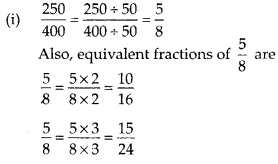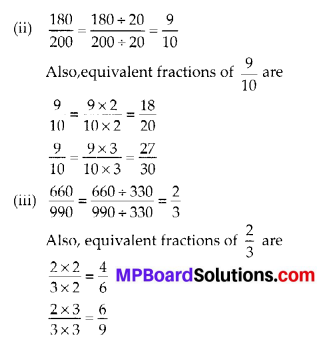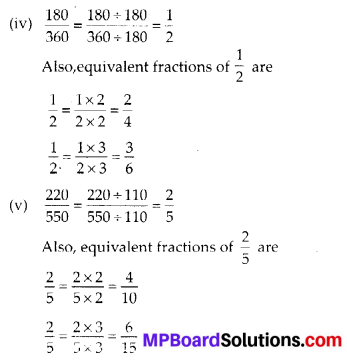## MP Board Class 6th Maths Solutions Chapter 7 Fractions Ex 7.3

Question 1.
Write the fractions. Are all these fractions equivalent?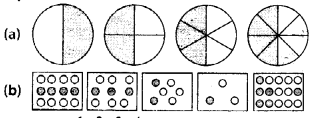Solution:
(a) $$\frac{1}{2}, \frac{2}{4}, \frac{3}{6}, \frac{4}{8}$$
Yes, all of these fractions are equivalent.

(b)
$$\frac{4}{12}, \frac{3}{9}, \frac{2}{6}, \frac{1}{3}, \frac{6}{15}$$
No, all these fractions are not equivalent.Question 2.
Write the fractions and pair up the equivalent fractions from each row.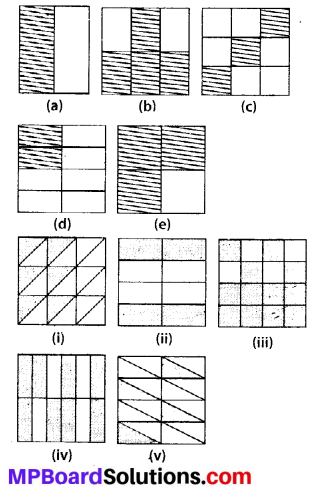Solution: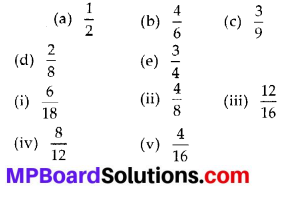Pairs of equivalent fractions are:
(a), (ii);
(b), (iv);
(c), (i);
(d), (v);
(e), (iii)

Question 3.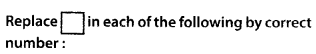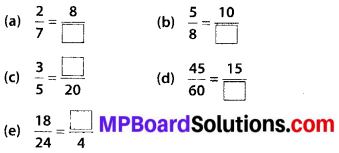Solution: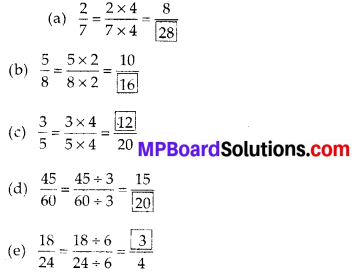Question 4.
Find the equivalent fraction of $$\frac{3}{5}$$ having
(a) denominator 20
(b) numerator 9
(c) denominator 30
(d) numerator 27
Solution: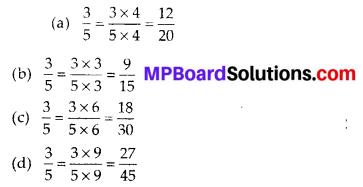Question 5.
Find the equivalent fraction of $$\frac{36}{48}$$ with
(a) numerator 9
(b) denominator 4.
Solution: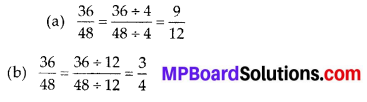Question 6.
Check whether the equivalent: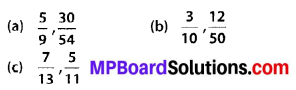Solution: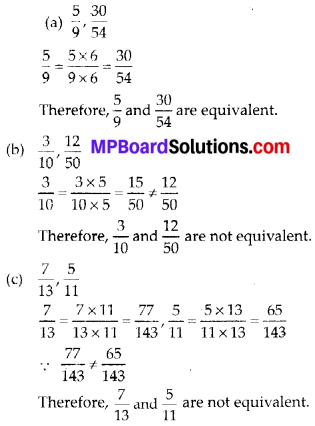Question 7.
Reduce the following fractions to simplest form: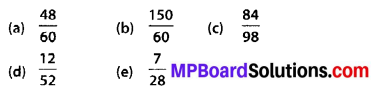Solution: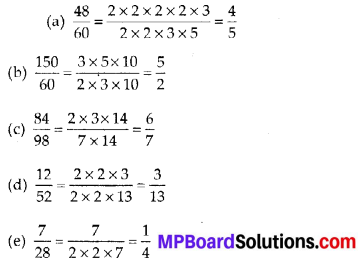Question 8.
Ramesh had 20 pencils, Sheelu had 50 pencils and Jamaal had 80 pencils. After 4 months, Ramesh used up 10 pencils, Sheelu used up 25 pencils and Jamaal used up 40 pencils. What fraction did each use up? Check if each has used up an equal fraction of her/his pencils?
Solution:
Ramesh : Total pencils = 20
Pencils used = 10
Fraction = $$\frac{10}{20}=\frac{1}{2}$$
Sheelu : Total pencils = 50
pencils used = 25
Fraction = $$\frac{25}{50}=\frac{1}{2}$$
Jamaal : Total pencils = 80
pencils used = 40
Fraction = $$\frac{40}{80}=\frac{1}{2}$$
Since, all of them used half of their pencils, therefore, each has used up equal fraction of pencils.Question 9.
Match the equivalent fractions and write two more for each. 250 2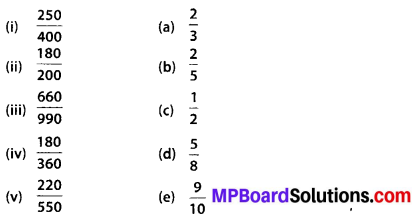Solution:
(i) ➝ (d);
(ii) ➝ (e);
(iii) ➝ (a);
(iv) ➝ (c);
(v) ➝ (b)